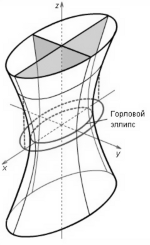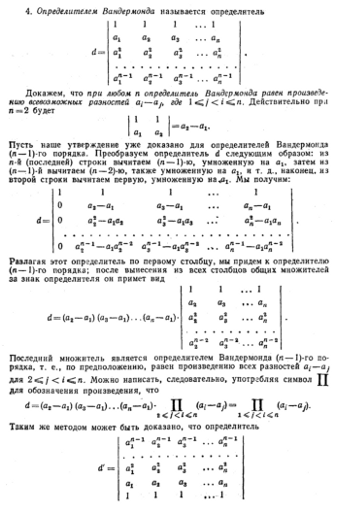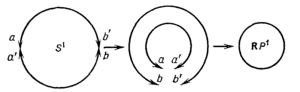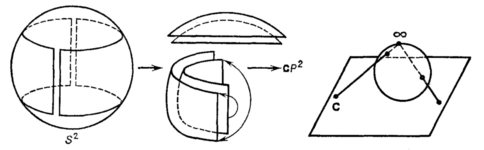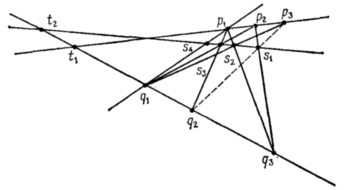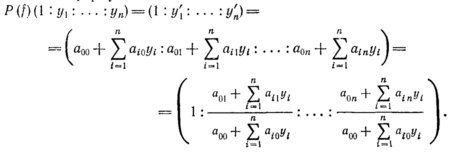# Peter Kogut's Major Publications

## Selected papers (listed in reverse chronological order)

#### 2019

1. P.I. Kogut, O.P. Kupenko, On Optimal Control Problem for an Ill-Posed Strongly Nonlinear Elliptic Equation with $p$-Laplace Operator and $L^1$-Type of Nonlinearity , Discrete and Continuous Dynamical Systems, Series B, Vol.24, Issue 3, 2019, 1273–1295. [Show Abstract]
2. P.I.Kogut, O.P. Kupenko, On Approximation of an Optimal Control Problem for Ill-Posed Strongly Nonlinear Elliptic Equation with $p$-Laplace Operator, in "Modern Mathematics and Mechanics. Fundamentals, Problems and Challenges", Editors: Sadovnichiy, Victor A., Zgurovsky, Michael (Eds.), Springer, 2019, Chapter 23, 445-480. [Show Abstract]
3. C. D'Apice, U. De Maio, P.I. Kogut, An Indirect Approach to the Existence of Quasi-Optimal Controls in Coefficients for Multi-Dimensional Thermistor Problem, Networks and Homogeneous Media, 2019, (to appear). [Show Abstract]
4. U. De Maio, P.I. Kogut, G. Zecca, On Optimal $L^1$-Control in Coefficients for Quasi-Linear Dirichlet Elliptic Boundary Value Problem with $BMO$-Anisotropic $p$-Laplacian, Mathematical Control and Related Fields, 2019, (submitted). [Show Abstract]
5. P.I. Kogut, On Optimal and Quasi-Optimal Controls in Coefficients for Multi-Dimensional Thermistor Problem with Mixed Dirichlet-Neumann Boundary Conditions , Control and Cybernetics, 2019 (to appear).
6. C. D'Apice, U. De Maio, P.I. Kogut, Optimal Boundary Control Problem for Ill-Posed Elliptic Equation in Domains with Rugous Boundary. I. Existence Result and Optimality Conditions, Optimal Control, Applications and Methods, 2019 (submitted).
7. C. D'Apice, P.I. Kogut, R. Manzo, Optimal Boundary Control Problem for Ill-Posed Elliptic Equation in Domains with Rugous Boundary. II. Asymptotic Analysis, Optimal Control, Applications and Methods, 2019 (submitted).

#### 2018

1. P.I.Kogut, O.P. Kupenko, On Approximation of State-Constrained Optimal Control Problem in Coefficients for $p$-Biharmonic Equation, J. of Optimization, Differential Equations and Their Applications (JODEA), Vol.26, Issue 1, 2018, 45-71. [Show Abstract]
2. C. D'Apice, M.-P. D'Arienzo, P.I. Kogut, R. Manzo, On Boundary Optimal Control Problem for an Arterial System: Existence of Feasible Solutions, Journal of Evolution Equations, 2018, Vol.18, Issue 4, 1745–1786. [Show Abstract]

#### 2016

1. T. Horsin, P. Kogut, On unbounded optimal controls in coefficients for ill-posed elliptic Dirichlet boundary value problems , Asymptotic Analysis, Volume 98, 2016, 155-188. [Show Abstract]
2. E. Casas, P.I. Kogut, G. Leugering, Approximation of Optimal Control Problems in the Coefficient for the $p$-Laplace Equation. I. Convergence Result, SIAM J. on Control and Optimization, 54(3), 2016, 1406-1422. [Show Abstract]
3. T. Horsin, P. Kogut, O. Wilk, : Optime L2-Control Problem In Coefficients For A Linea Elliptic Equation. II. Approximation Of Solutions And Optimality Conditions , Mathematical Control and Related Fields, Vol. 6, No.4, 2016, 595-628. [Show Abstract]
4. C. D’Apice; P.I. Kogut; R. Manzo, On Optimization of a Highly Re-Entrant Production System. Networks and Heterogeneous Media, Vol 11, Issue 3, September 2016. p.415-445. [Show Abstract]
5. P.I.Kogut, O.P. Kupenko, Optimality Conditions for $L^1$-Control in Coefficients of a Degenerate Nonlinear Elliptic Equation, in "Advances in Dynamical Systems and Control", Chapter 24, Springer, 2016, 429-469. [Show Abstract]
6. P.I. Kogut, G. Leugering, R. Schiel On Henig Regularization of Material Design Problems for Quasi-Linear p-Biharmonic Equation, Applied Mathematics, 7, 2016, 1547-1570 (Published Online August 2016 in SciRes. http://www.scirp.org/journal/am). [Show Abstract]
7. P.I. Kogut; R. Manzo; A.O. Putchenko, On approximate solutions to the Neumann elliptic boundary value problem with non-linearity of exponential type. Boundary Value Problems, Vol 2016, Issue 1, December 2016. p.1-32 (doi:10.1186/s13661-016-0717-1). KMP20161186 [Show Abstract]
8. P.I. Kogut, G. Leugering, R. Schiel , On the relaxation of state-constrained linear control problems via Henig dilating cones , Control and Cybernetics, Vol. 45, No. 2, 2016, p. 131–162. [Show Abstract]

#### 2015

1. P. I. Kogut, G. Leugering, Optimal and Approximate Boundary Control of an Elastic Body with Quasistatic Evolution of Damage, Mathematical Methods in the Applied Sciences, Volume 38, Issue 13, pages 2739–2760, 15 September 2015. [Show Abstract]
2. P. I. Kogut, O. P. Kupenko, G. Leugering, Optimal Control Problems in Coefficients for Nonlinear Dirichlet Problems of Monotone Type: Optimality Conditions. Part I , Journal of Analysis and its Applications (ZAA), Volume 34, Issue 1, 2015, 85-108. KKL1_2015PDF [Show Abstract]
3. P. I. Kogut, O. P. Kupenko, G. Leugering, Optimal Control Problems in Coefficients for Nonlinear Dirichlet Problems of Monotone Type: Optimality Conditions. Part II , Journal of Analysis and its Applications (ZAA), Volume 34, Issue 2, 2015, 199-219. KKL2_2015PDF [Show Abstract]
4. T. Horsin, P. Kogut, Optimal L^2-Control Problem in Coefficients for a Linear Elliptic Equation. I. Existence Result , Mathematical Control and Related Fields, Volume 5, Number 1, March 2015, 73-96. KT_MCRF_1PDF [Show Abstract]

#### 2014

1. A. V. Dovzhenko, P. I. Kogut, R. Manzo, Epi and Coepi-Analysis of Vector-Valued Mappings, Optimization. A Journal of Mathematical Programming and Operations Research, 2014, Vol 63, Issue 4, P. 535-557. [Show Abstract]
2. P.I. Kogut, R.Manzo, On Quadratic Scalarization of Vector Optimization Problems in Banach Spaces , Applicable Analysis, Vol. 93, No. 5, 2014, 994-1009. F2.2013PDF [Show Abstract]
3. C. D'Apice, U. De Maio, P. I. Kogut, R. Manzo, On the Solvability of an Optimal Control Problem in Coefficients for Ill-Posed Elliptic Boundary Value Problems, Electronic Journal of Differential Equations, Vol. 2014, No. 166, 2014, 1-23. [Show Abstract]
4. P.I. Kogut, On Approximation of an Optimal Boundary Control Problem for Linear Elliptic Equation with Unbounded Coefficients, to appear in Discrete and Continuous Dynamical Systems - Series A (DCDS-A), Vol.34, No.5, 2014, 2105-2133. [Show Abstract]
5. P. I. Kogut, G. Leugering, On Existence of Optimal Solutions to Boundary Control Problem for an Elastic Body with Quasistatic Evolution of Damage, In the book "Continuous and Distributed Systems: Theory and Applications", Series: Solid Mechanics and Its Applications, Springer-Verlag, Berlin, 2014, Vol.211, Chapter 19, p.265-286. KL_2013_1PDF [Show Abstract]
6. C. D'Apice, P. I. Kogut, R. Manzo, On Relaxation of State Constrained Optimal Control Problem for a PDE-ODE Model of Supply Chains, Networks and Heterogeneous Media, Volume 9, Issue 3, 2014, Pages 501-518. pdfsPDF [Show Abstract]

#### 2013

1. P. I. Kogut, G. Leugering, Matrix-valued L1-optimal control in the coefficients of linear elliptic problems, ZAA (Zeitschrift für Analysis und ihre Anwendungen), Volume 32, Issue 4, 2013, p. 433–456. F3.2013PDF [Show Abstract]
2. P.I. Kogut, R.Manzo, On Vector-Valued Approximation of State Constrained Optimal Control Problems for Nonlinear Hyperbolic Conservation Laws , Journal of Dynamical and Control Systems, No.2, Vol.19, 2013, 381-404. F1.2013PDF [Show Abstract]
3. T. Horsin, P. Kogut, Optimal L^2-Control Problem in Coefficients for a Linear Elliptic Equation, {submitted to Mathematical Control and Related Fields}, 2013, 1-60, arXiv:1306.2513. [Show Abstract]

#### 2012

1. P. I. Kogut, G. Leugering, Optimal L1-Control in Coefficients for Dirichlet Elliptic Problems: H-Optimal Solutions, Zeitschrift für Analysis und ihre Anwendungen, (2012), Vol.31, Issue 1, 31-53. [Show Abstract]
2. І. Г. Баланенко, П. І. Когут, Про одну задачу оптимального керування для виродженого параболічного рівняння, Вісник ДНУ. Сер. Моделювання, № 8, Вип. 4, 2012. — С. 3–18. [Show Abstract]
3. P.I.Kogut, O.P.Kupenko, On Attainability of Optimal Solutions for Linear Elliptic Equations with Unbounded Coefficients, Вісник ДНУ. Сер. Моделювання, № 8, Вип. 4, 2012. — С. 63–82. [Show Abstract]

#### 2011

1. A. В. Довженко, П. I. Когут, Epi-напівнеперервні знизу відображення та їх властивості, Математичні студії, 36(1) 2011, 86-96. KD_2011PDF [Show Abstract]
2. G. Buttazzo, P. I. Kogut, Weak optimal controls in coefficients for linear elliptic problems, Revista Matematica Complutense, Vol.24, 2011, pp. 83–94. file_2PDF [Show Abstract]
3. A.V. Dovzhenko, P.I. Kogut, R. Manzo, On the concept of Г-convergence for locally compact vector-valued mappings, Far East Journal of Applied Mathematics, Vol.60, Issue 1, 2011, p.1-39. [Show Abstract]
4. P. I. Kogut, G. Leugering, Optimal L1-Control in Coefficients for Dirichlet Elliptic Problems: W-Optimal Solutions, Journal of Optimization Theory and Applications, 150(2) (2011), 205-232. [Show Abstract]
5. П. И. Когут, К вопросу об устойчивости Lp-решений интегральных уравнений Вольтерра, Вісник ДНУ. Сер. Моделювання, Т.19, № 8, Вип. 3, 2011. — С. 3–19. Kogut_1_11PDF [Show Abstract]

#### 2010

1. P. I. Kogut, R. Manzo, I. V. Nechay, Generalized efficient solutions to one class of vector optimization problems in Banach spaces, Australian Journal of Mathematical Analysis and Applications, 2010, Vol.7, Issue 1., pp. 1–27. [Show Abstract]
2. P. I. Kogut, Manzo R., I. V. Nechay, Topological Aspects of Scalarization in Vector Optimization Problems, Australian Journal of Mathematical Analysis and Applications, 2010, Vol.7, Issue 2., pp. 25–49. [Show Abstract]
3. C. D'Apice, P. I. Kogut, R. Manzo, Efficient controls for one class of fluid dynamic models, Far East Journal of Applied Mathematics, 2010, Vol.46, No.2, pp. 85–119. [Show Abstract]
4. P. I. Kogut, Rudyanova T.N. On some smooth approximations on thick periodic multi-structures, Вісник ДНУ. Сер. Математика, Т.18, № 6/1, Вип. 15, 2010. — С. 158–170. Kogut_1_15PDF [Show Abstract]
5. C. D'Apice, P. I. Kogut, R. Manzo, Efficient Controls for Traffic Flow on Networks, Dynamical and Control Systems, 2010, Vol.16, No.3, pp. 407–437. Kogut_1_16PDF [Show Abstract]
6. P. I. Kogut, Manzo R., I. V. Nechay, On existence of efficient solutions to vector optimization problems in Banach spaces, Note di Matematica, Vol.30(1), 2010, pp. 25–39. Kogut_1_17PDF [Show Abstract]
7. C. D'Apice, P. I. Kogut, R. Manzo, On Approximation of Entropy Solutions for One System of Nonlinear Hyperbolic Conservation Laws with Impulse Source Terms, Journal of Control Science and Engineering, 2010, Vol. 46, No. 2. p.85-119. Kogut_1_18PDF [Show Abstract]

#### 2009

1. V. V. Gotsulenko, P. I. Kogut, Suboptimal boundary control of the Benard system in a cylindrically perforated domain, Ukrainian Mathematical Bulletin, Vol. 6, No. 4, 2009, pp. 431–467. Kogut_1_20PDF [Show Abstract]
2. I. G. Balanenko, P. I. Kogut, On existence of the weak optimal BV-controls in coefficients for linear elliptic problems, Вісник ДНУ. Сер. Моделювання, Т.17, № 8, Вип. 1, 2009. — С. 93–103. [Show Abstract]
3. P. I. Kogut, G. Leugering, Homogenization of optimal control problems for one-dimensional elliptic equations on periodic graph, ESAIM: Control, Optimization and Calculus of Variations (COCV), Vol. 15, 2009, pp. 471–498. Kogut_1_23PDF [Show Abstract]
4. C. D'Apice, U. De Maio, P. I. Kogut, Boundary velocity suboptimal control of incompressible flow in cylindrically perforated domain, Discrete and Continuous Dynamical Systems, Serie B, Vol.11, No.2, 2009, pp. 283–314. Kogut_1_22PDF [Show Abstract]
5. А. В. Довженко, П. И. Когут, Квазі-неперервна знизу регуляризація відображень в бананових просторах, Вісник ДНУ, Сер. Математика, Т.17, № 6/1, Вип. 14, 2009. — С. 60–72. [Show Abstract]

#### 2008

1. C. D'Apice, U. De Maio, P. I. Kogut, Gap phenomenon in homogenization of parabolic optimal control problems, IMA Journal of Mathematical Control and Information (Cambridge University), Vol.25, 2008, pp. 461–480. file_2_01PDF [Show Abstract]
2. C. D'Apice, U. De Maio, P. I. Kogut, Suboptimal boundary controls for elliptic equations in critically perforated domains, Annales de I'Institut Henri Poincare (C), Nonlinear Analysis, Vol.25, Issue 6, 2008, pp. 1073–1101. file_2_02PDF [Show Abstract]
3. P. I. Kogut, G. Leugering, Homogenization of Dirichlet optimal control problems with exact partial controllability constraints, Asymptotic Analysis, Vol.57, No.3-4, 2008, pp. 229–249. file_2_03PDF [Show Abstract]
4. P. I. Kogut, I. V. Nechay, On scalarization of vector optimization problems in Banach spaces, J. of Automation and Information Sciences, Vol.40, Issue 12, 2008, pp. 1–13. file_2_04PDF [Show Abstract]
5. P. I. Kogut, T. N. Rudyanova, On the weakly-∗ dense subsets in L(Ω), Вісник ДНУ, Сер. Математика, Т.16, №6/1, 2008, pp. 149–154. file_2_05PDF [Show Abstract]

#### 2007

1. P. I. Kogut, G. Leugering, Asymptotic analysis of state constrained semilinear optimal control problems, Journal of Opt. Theory and Appl. (JOTA), Vol.135, No.2, 2007, pp. 301–321. file_2_07PDF [Show Abstract]
2. P. I. Kogut, G. Leugering, On the homogenization of optimal control problems on periodic graphs, Lecture Notes in Pure and Applied Mathematics, Vol.252, 2007, pp. 55–74. file_2_08PDF [Show Abstract]
3. П. І. Когут, М. Є. Сердюк, Задачі каркасної інтерполяції статичних зображень, Вісник Херсонського техн. унів., № 2(28), 2007, 153–157.
4. P. I. Kogut, T. N. Rudyanova, On the property of homothetic mean value on periodically perforated domains, Вісник ДНУ, Сер. Математика, №8, 2007, 158–166. file_2_09PDF [Show Abstract]
5. П. І. Когут, М. Є. Сердюк, Про задачу реконструкції зображень за їх каркасною інтерполяцією, Системні технології, №3(50), 2007, 35–45.

#### 2006

1. П. І. Когут, І. В. Нечай, Про розвязність одного классу задач векторної оптимізації, Наукові Вісті ННТУ-КПІ, № 5, 2006, C. 148–158.
2. P. I. Kogut, V. M. Mizerny, T. M. Rudjanova, On homogenization of optimal control problems. I. Analysis of existing approaches, J. Automation and Information Sciences, 38(2006), i. 5, 6-22; translated from Problemy Upravlenija I Informatiki No.3, 2006, pp. 10–26.
3. P. I. Kogut, V. M. Mizerny, T. M. Rudjanova, On homogenization of optimal control problems. II. Variational convergence of the constrained minimization problems, J. Automation and Information Sciences, 38, i. 7, 2006, pp. 58–72; translated from Problemy Upravlenija I Informatiki No. 4, 58-71(2006).

#### 2005

1. П. И. Когут, Т. А. Мельник, К усреднению одной задачи оптимального управления в густых сингулярных соединениях, Доп. НАН України, 2005, N.7. — C.52–58;
2. P. I. Kogut, T. A. Mel'nik, Limit analysis of one class of optimal control problems in thick singular junctions, J. Automation and Information Sciencis, Vol. 37, i. 1, 2005, 8–23; translated from Problemy Upravlenija I Informatiki No.2, 13-31(2005).
3. P. I. Kogut, G-convergence of nonlinear operators in the theory of homogenization of control objects, Cybernetics and System Analysis, Vol. 41, No 5, 2005, 670–687; translated from Kibern. Sist. Anal., No.5, 42-65 (2005).

#### 2004

1. Н. А. Баламут, П. И. Когут, Об усреднении одной нелинейной задачи оптимального управления, Доп. НАН України, 2004, N. 2. — C. 60–65.
2. P. I. Kogut, T. A. Mel'nik, Asymptotic analysis of optimal control problems in thick multi-structures, In the book "Generalized Solutions in Control Problems", Proceeding of the IFAC Workshop GSCP-2004, Pereslavl-Zalessky, Russia, September 21-29,2004. General Theory: Distributed Parameter Systems, p. 265–275. file_2_10DVI

#### 2003

1. Н. А. Баламут, П. И. Когут, Предельный анализ одного класса квазилинейных задач оптимального управления с фазовыми ограничениями, Проблемы управления и информатики, 2003, N.4. — C. 29–43; English trans. in J. Automatation and Information Sciences, 2003, V. 35, N 7, 25-38.
2. P. I. Kogut, V. N. Mizernuy, T. N. Rudyanova, On homogenization of cotrolled objects described by operators equation of Gammerstain type, J. Automation and Information Sciences, 35(2003), i. 11, pp. 19–28; translated from Problemy Upravlenija I Informatiki No.6, 24–34(2003).
3. N. A. Balamut, P. I. Kogut, Limit analysis of one class of quasilinear problems of optimal control with constraints on the state, J. Automation and Information Sciences, 35(2003), i. 7, pp. 25–38; translated from Problemy Upravlenija I Informatiki No.4, 29–43(2003).

#### 2002

1. П. И. Когут, В. Н. Мизерный, Об усреднении экстремальных задач для линейных операторных уравнений Гаммерштейна, Доп. НАН України, 2002, N. 4. — C. 66–71.
2. P. I. Kogut, G. Leugering, S-homogenization of optimal control problems in Banach spaces, Mathematische Nachrichten, 2002, V.233-234, pp. 141–169.

#### 2001

1. P. I. Kogut, G. Leugering, On S-homogenization of an optimal control problem with control and state constraints, ZAA (J. Mathematical Methods in the Appl. Sci.), 2001, V. 20, No.2, pp. 395–429.
2. P. I. Kogut, G. Leugering, Homogenization of optimal control problems in variable domains. Principle of fictitious homogenization, J. Asymptotic Analysis, 2001, V.26, pp. 37–72.
3. P. I. Kogut, O. V. Museyko, On the S-Homogenization of Variational Inequalities in Banach Spaces, Доповiдi НАН України, 2001, N 5. — С. 73–76.
4. P. I. Kogut, On the topological convergence of sets in partially ordered spaces, Нелинейные граничные задачи, 2001, No.11. — C. 94–97.
5. П. И. Когут, В. Н. Мизерный, К усреднению оптимизационных задач для операторных уравнений Гаммерштейна, Проблемы управления и информатики, 2001, N.6. — C. 37–53; English transl. in J. Automatation and Information Sciences, 2001, V. 33, N. 11, pp. 36–50.
6. P. I. Kogut, V. S. Mel'nyk, On the weak compactness of bounded sets in Banach and loccally convex spaces, Ukrainian Mathematical Journal, 52(2001), No. 6, pp. 837–846; translated from Ukr Mat. Zh. Vol.52, No.6, 731-739(2000).
7. P. I. Kogut, O. V. Museyko, On S-homogenization of linear variational inequalities in Banach spaces. Regular case, J. Automation and Information Sciences, 35(2001), I. 9, pp. 31-42; translated from Problemy Upravlenija I Informatiki No.5, 27–40(2001).
8. P. I. Kogut, V. N. Mizernuy, On homogenization of optimization problems for operators equations of the Gammersain type, J. Automation and Information Sciences, 33(2001), i. 11, pp. 36–50; translated from Problemy Upravlenija I Informatiki No.6, 37–53(2001).

#### 2000

1. П. И. Когут, В. С. Мельник, О слабой компактности ограниченных множеств в банаховых и локально выпуклых пространствах, Укр. математ. журн., 2000, Т. 52, N 6. — С. 731–739; English transl. in Ukrainian Mathematical Journal, 2001, V. 52, No. 6, pp. 837–846.
2. П. И. Когут, О. В. Мусейко, Об одном подходе к усреднению нелинейных вариационных неравенств в банаховых пространствах, Сложные системы управления, 2000, T. 129.— С. 18–28.
3. P. I. Kogut, Moro-Yosida approximation of conditional minimization problems and its limit properties, J. Math. Sci., New York 102(2000), No. 6, pp. 3775–3781; translated from Obchisl. Prikl. Mat. 81, 62–69 (1997).

#### 1999

1. P. I. Kogut, On the structural representation of S-homogenized optimal control problems, Nonlinear Boundary Value Problems, 1999, V. 9. – pp. 84–91.
2. П. И. Когут, Про топологiчну збiжнiсть надграфiкiв в частково впорядкованих векторних просторах, Математичнi студiї, 1999, N 12. — С. 161–170.

#### 1998

1. P. I. Kogut, V. S. Mel'nik, On one class of extremum problems for nonlinear operator systems, Cybern. Syst. Anal., Vol. 34, 1998, No.6, pp.894–904; translated from Kibern. Sist. Anal. No.6, 121-132 (1998).
2. П. И. Когут, О конструировании метрических пространств на множестве задач условной минимизации, Проблемы управления и информатики, 1998, N 1. – С. 37–47.
3. П. И. Когут, О Γ-представлении вариационных S-пределов задач условной минимизации в нормальных хаусдорфовых пространствах, Кибернетика и системный анализ, 1998, N 1. – С. 104–118; English transl. in Cybern. Syst. Anal., 1998, V. 34, No. 1, pp. 86–96.
4. P. I. Kogut, On averaging of a singularly perturbed optimal control problem, Cybernetics and Computing Technology, Discrete Control Systems, Vol. 113, 1998, pp. 59–72 ; translated from Kibernetika i Vitchislitelnaja Technika, N.113, 67–81(1998).

#### 1997

1. П. I. Когут, Варiацiйна S-збiжнiсть задач мiнiмiзацiї та її геометрична iнтерпретацiя, Доп. НАН України, 1997, N 6. – С. 89–93.
2. P. I. Kogut, S-convergence in the theory of homogenization of problems of optimal control, Ukrainian Mathematical Journal, Vol. 49, 1997, No. 11, pp. 1671-1682; translated from Ukr Mat. Zh., Vol.49, No.11, 1488-1498(1997).
3. A. I. Egorov, P. I. Kogut, Homogenization of Optimal Control Problems in Coeffitients for Linear Elliptic Equations, J. of . Autom. Information Sci., Vol. 29, 1997, No. 4-5, pp. 37–46; ; translated from Problemy Upravlenija I Informatiki, No.6, 89–104(1995).

#### 1996

1. П. И. Когут, Асимптотичний аналiз керованих параболiчних систем з еволюцiєю на границi, Доповiдi НАН України, 1996, N 10. – С. 99–103.
2. P. I. Kogut, High-order asymptotics og the solution of a problem of optimal control of a distributed system with rapidly oscillating coefficients, Ukr. Math. J., Vol. 48, 1996, N. 7, pp. 1063–1073; translated from Ukr Mat. Zh., Vol.48, No.7, 940–948(1996).
3. P. I. Kogut, Variational convergence of optimal control problems for elliptic systems, J. Automation and Information Sciences, Vol. 28, 1996, N. 3-4, pp. 1063–1073; translated from Problemy Upravlenija I Informatiki, No.3, 72–84(1995).

#### 1995

1. П. И. Когут, Вариационная сходимость задач оптимального управления для систем эллиптического типа, Проблеми управления и информатики, 1995, N 3. – С. 72–84.
2. А. И. Егоров, П. И. Когут, Усреднение задач оптимального управления коэффициентами в линейных эллиптических уравнениях, Проблемы управления и информатики, 1995, N 6. – С. 89–104.
3. P. I. Kogut, On averaging of a singularly perturbed optimal control problem, Cybernetics and Computing Technology discrete control systems, 1995, N 113. — pp. 59–72.
4. P. I. Kogut, State exponential stability of a denumerable system of the Volterra integral equations, J. Automation and Information Sciencis, Vol. 27, 1995, No.5-6, pp. 60–75; translated from Avtomatika No.5, 38-47 (1991).

#### 1994

1. A. I. Egorov, P. I. Kogut, On the state stability of a system of integro-differential equations of nonstationary aeroelasticity, J.of Mathematical Sciences (Springer, New York), Vol. 70, 1994, N. 1, pp. 1578–1585 ; translated from Vychislitel'naja i Prikladnaja Matematika, N.70, 112–121(1990).

#### 1991

1. P. I. Kogut, State exponential stability of a denumerable system of the Volterra integral equations, Sov. J. Autom. Inf. Sci., Vol. 24, 1991, No. 5, pp. 37–46 ; translated from Avtomatika, No.5, 38–47 (1991).

#### 1990

1. П. И. Когут, Об экспоненциальной устойчивости по состоянию счетной системы интегральных уравнений Вольтерра, Автоматика, 1990, N 5. – С.  38–47; English transl. in J. of Automation and Information Sciences, 1995, Vol.  27, N 5–6. — P. 60–75 and in Sov. J. Autom. Inf. Sci., 1991, V. 24, No.5, 37–46.

#### 1989

1. А. И. Егоров, П. И. Когут, Оптимальный синтез для уравнений нейтрального типа, Докл. АН УССР, Сер.А, 1989, N 5. – С. 64–67.